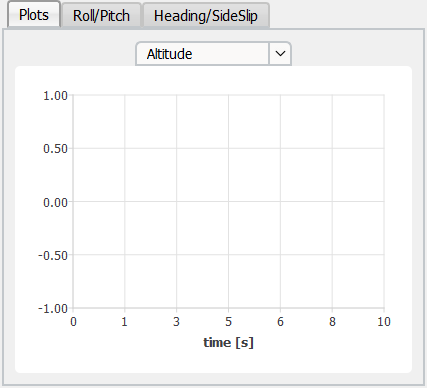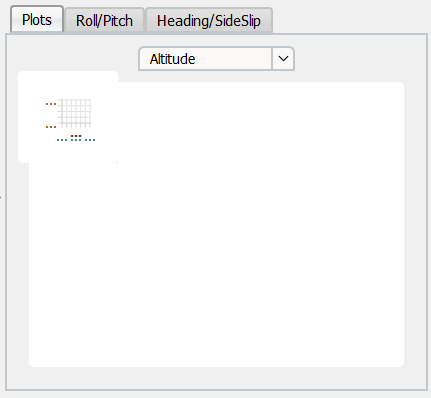# Problem with data visualization in QCharts and multithreading

• Hello everyone.
I got a problem regarding QChartView and widgets in general when used with multithreading.

I got a code like this

``````QChart         *chart_plot;
QChartView     *chartView_plot;
QLineSeries    *series_plot;
``````

in my mainwindow.h and like this

``````series_plot = new QLineSeries();
chart_plot = new QChart();

// etc...

chartView_plot = new QChartView;
chartView_plot->setChart(chart_plot);
chartView_plot->setRenderHint(QPainter::Antialiasing,true);

``````

in my mainwindow.cpp ant it works fine with the following result:However, since there are more charts in my GUI and everything starts beeing slower and less reactive, I was thinking about letting another thread to manage the data visualization.

``````QChart         *chart_plot;
QChartView     *chartView_plot;
QLineSeries    *series_plot;
``````

``````void MyThread_navigation::setup_thread(QThread &cThread, QChartView *chartview)
{
series_plot = new QLineSeries();
chart_plot = new QChart();

// etc...

chartView_plot = new QChartView(chartview);
chartView_plot->setChart(chart_plot);
chartView_plot->setRenderHint(QPainter::Antialiasing,true);

}
``````

Then, I wrote

``````MyThread_navigation *worker_navigation;           // Worker for navigation data visualization
``````

in mainwindow.h and this

``````chartView_plot = new QChartView();
chartView_plot->setRenderHint(QPainter::Antialiasing,true);

``````

in mainwindow.cpp.

The result is the following:Moreover, when I resize the GUI window, that small chart does not appear to change its size.
It seems that when I pass a widget to another class and move that class to my QThread object, my chart won't resize to the layout's size in which it is contained.

In previous codes I wrote something like

``````graph_surface = new Q3DSurface();
container     = QWidget::createWindowContainer(graph_surface);

graph_surface->setHorizontalAspectRatio(container->width()/container->height());
graph_surface->setHeight(container->height());

container->setSizePolicy(QSizePolicy::Expanding, QSizePolicy::Expanding);
container->setFocusPolicy(Qt::StrongFocus);

// etc.

``````

and It used to work fine. The problem is that `createWindowContainer` doesn't take a QChartView as a input (only QWindow apparently).
How can I solve this?
How can I "put" a QChartView (QWidget) in a container (if it solves my problem)?
Is there a better way to make another thread to manage data visualization on a chart?